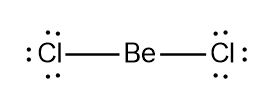# Problem: Classify each of the following as a Lewis acid or a Lewis base.BeCl2, NH3, O2-, SO3, Fe2+

###### FREE Expert Solution
95% (464 ratings)
###### FREE Expert Solution
• Recall that a Lewis acid is the one accepting an electron pair while a Lewis base is the one donating

• For BeCl2, if we will analyze its Lewis structure, Be will have 2 valence ewhile Cl will have 7 electrons, total of 16. Be will appear to be single bonded to both Cl atoms.95% (464 ratings)###### Problem Details

Classify each of the following as a Lewis acid or a Lewis base.

BeCl2, NH3, O2-, SO3, Fe2+

What scientific concept do you need to know in order to solve this problem?

Our tutors have indicated that to solve this problem you will need to apply the Lewis Acid and Base concept. You can view video lessons to learn Lewis Acid and Base. Or if you need more Lewis Acid and Base practice, you can also practice Lewis Acid and Base practice problems.

What is the difficulty of this problem?

Our tutors rated the difficulty ofClassify each of the following as a Lewis acid or a Lewis ba...as medium difficulty.

How long does this problem take to solve?

Our expert Chemistry tutor, Sabrina took 6 minutes and 4 seconds to solve this problem. You can follow their steps in the video explanation above.

What professor is this problem relevant for?

Based on our data, we think this problem is relevant for Professor Badjic's class at OSU.